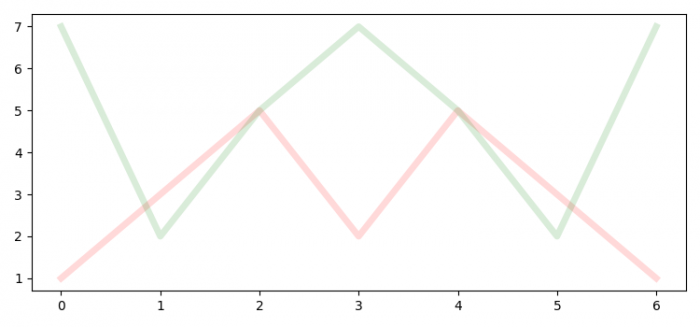# How to plot overlapping lines in Matplotlib?

To plot overlapping lines in matplotlib, we can use variable overlapping that basically sets the opacity or alpha value in the plot.

## Steps

• Set the figure size and adjust the padding between and around the subplots.
• Initialize a variable overlapping to set the alpha value of the line.
• Plot line1 and line2 with red and green colors, respectively, with the same alpha value.
• To display the figure, use show() method.

## Example

from matplotlib import pyplot as plt
plt.rcParams["figure.figsize"] = [7.50, 3.50]
plt.rcParams["figure.autolayout"] = True
overlapping = 0.150
line1 = plt.plot([1, 3, 5, 2, 5, 3, 1], c='red', alpha=overlapping, lw=5)
line2 = plt.plot([7, 2, 5, 7, 5, 2, 7], c='green', alpha=overlapping,
lw=5)
plt.show()

## Output